# Large Amplitude Pendulum

 The usual solution for the simple pendulum depends upon the approximation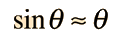which gives the equation for the angular accelerationbut for angles for which that approximation does not hold, one must deal with the more complicated equation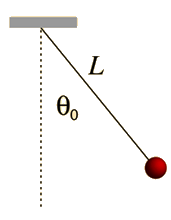The detailed solution leads to an elliptic integral. That solution can be approximated by a series, for which the first three terms are### Calculation

Index

Periodic motion concepts

 HyperPhysics***** Mechanics R Nave
Go Back

# Large Amplitude Pendulum

An approximation for the large amplitude pendulum period
 For L = cm and g = m/s2
 when swung from angle θ0 = degrees: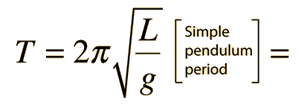s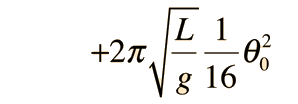s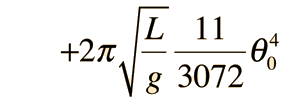s for approximate period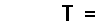sThis period deviates from the simple pendulum period by percent. You can explore numbers to convince yourself that the error in pendulum period is less than one percent for angular amplitudes less than 22 degrees.
Index

Periodic motion concepts

 HyperPhysics***** Mechanics R Nave
Go Back